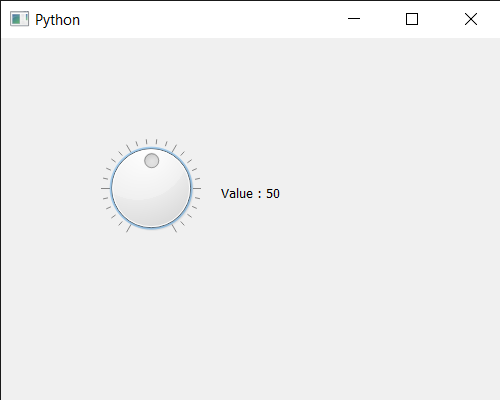# PyQt5 QDial – Getting Slider’s Value

In this article we will see how we can get slider’s value of the QDial. User can change the value with the help of mouse although we can change it programmatically with the help of `setValue` method. The slider forces the value to be within the legal range i.e value should be greater than or equal to the minimum value and it should be less than or equal to the maximum limit. Changing the value also changes the slider position.

In order to do this we use `value` method with the QDial object

Syntax : dial.value()

Argument : It takes no argument

Return : It returns integer

Below is the implementation

 `# importing libraries ` `from` `PyQt5.QtWidgets ``import` `*`  `from` `PyQt5 ``import` `QtCore, QtGui ` `from` `PyQt5.QtGui ``import` `*`  `from` `PyQt5.QtCore ``import` `*`  `import` `sys ` ` `  ` `  `class` `Window(QMainWindow): ` ` `  `    ``def` `__init__(``self``): ` `        ``super``().__init__() ` ` `  `        ``# setting title ` `        ``self``.setWindowTitle(``"Python "``) ` ` `  `        ``# setting geometry ` `        ``self``.setGeometry(``100``, ``100``, ``500``, ``400``) ` ` `  `        ``# calling method ` `        ``self``.UiComponents() ` ` `  `        ``# showing all the widgets ` `        ``self``.show() ` ` `  `    ``# method for components ` `    ``def` `UiComponents(``self``): ` ` `  `        ``# creating QDial object ` `        ``dial ``=` `QDial(``self``) ` ` `  `        ``# setting geometry to the dial ` `        ``dial.setGeometry(``100``, ``100``, ``100``, ``100``) ` ` `  `        ``# making notch visible ` `        ``dial.setNotchesVisible(``True``) ` ` `  `        ``# value ` `        ``value ``=` `50` ` `  `        ``# setting value to the dial slider ` `        ``dial.setValue(value) ` ` `  ` `  `        ``# creating a label ` `        ``label ``=` `QLabel(``"GeeksforGeeks"``, ``self``) ` ` `  `        ``# setting geometry to the label ` `        ``label.setGeometry(``220``, ``125``, ``200``, ``60``) ` ` `  `        ``# making label multiline ` `        ``label.setWordWrap(``True``) ` `         `  `        ``# getting slider value ` `        ``value ``=` `dial.value() ` `         `  `        ``# setting text to the label ` `        ``label.setText(``"Value : "` `+` `str``(value)) ` ` `  ` `  ` `  ` `  `# create pyqt5 app ` `App ``=` `QApplication(sys.argv) ` ` `  `# create the instance of our Window ` `window ``=` `Window() ` ` `  `# start the app ` `sys.exit(App.``exec``()) `

Output :My Personal Notes arrow_drop_upCheck out this Author's contributed articles.

If you like GeeksforGeeks and would like to contribute, you can also write an article using contribute.geeksforgeeks.org or mail your article to contribute@geeksforgeeks.org. See your article appearing on the GeeksforGeeks main page and help other Geeks.

Please Improve this article if you find anything incorrect by clicking on the "Improve Article" button below.

Article Tags :

Be the First to upvote.

Please write to us at contribute@geeksforgeeks.org to report any issue with the above content.